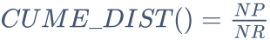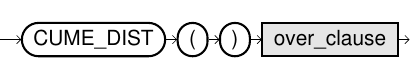# CUME_DIST

## Purpose

This function calculates the cumulative distribution of a value in a group of values. This is computed for each row as follows:where,

• NP is the number of rows with order value less than or equal to the current row
• NR is the total number of rows in the group

## Syntax

cume_dist::=## Usage Notes

• CUME_DIST is only available as an analytic function (i.e., in combination with an over_clause). For more information, refer to Analytic Functions section.
• This function returns values in the range (0, 1].
• CUME_DIST does not allow the use of a window_frame_clause. It uses the entire partition as window for the computation of the result.

## Example

### Analytic Function

``SELECT     id, department, current_salary,     CUME_DIST() OVER (PARTITION BY department ORDER BY current_salary) CUME_DIST FROM employee_table ORDER BY department, current_salary;``
###### Result
 ID DEPARTMENT CURRENT_SALARY CUME_DIST 2001 ACCOUNTS 55000 0.2 2002 ACCOUNTS 65000 0.4 2004 ACCOUNTS 70000 0.6 2003 ACCOUNTS 80000 1 2005 ACCOUNTS 80000 1 1001 HR 55000 0.25 1002 HR 70000 0.75 1004 HR 70000 0.75 1003 HR 90000 1